# Mathematics - Mathematical Analysis Limits and ContinuityHello its a me again! Today we continue with Mathematical Analysis getting into Limits and Continuity. The first two posts where about other things that have to do with functions and I suggest you to go and check them out if you haven't already, because we will use a lot of knowledge from there! So, without further do let's get into it!

## Introduction:

The monotony and extrema values of functions that we covered last time are a part of the Differential Calculus together with Limits and Derivatives. Differential Calculus is one of the 2 main branches of Calculus the other one being Integral Calculus, that contains the so called integrals in which we will get posts later on.

So, why do we need the differential calculus? Well, the applications in which derivatives and limits are used are endless. Think about Physics for example. The acceleration is the result of changing the velocity of a moving object that is related to the distance traveled in an time unit. Calculating limits and derivatives of functions we find rates of change of one unit to another unit that can be distance and time if we are talking about an Physics problem.

## Limits:

To get into all this stuff we first have to talk about the most essential part that are the limits. Suppose a function f and f(x) are the values of f for an indepentent variable x. The value "l" to which the values of f(x) are approaching when the value of x approaches a real number or +-infinity is called a limit.

The way we write it is as following: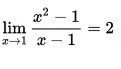This means that the values of f(x) when x approaches the number 1 approach the value 2.

If you try putting x = 1 in the function f(x) you see that we get a 0/0! Does this mean that 0/0 = 2? No, it doesn't. This is an indeterminate form for limits and needs another way of solving. But, yes sometimes we can directly put the value of x in the function and find the limit.

We have also another way of defining the limit, the so called epsilon-delta definiton that you can check out here and I think that talking about it may confuse you a little bit and so we stay with the simple definition for now!

A function has a limit to a number c when the limit is a real number, else it is non-existent and we say that the function has no limit to the value c.

Also, the limit doesn't have to apply to the whole Definition Set, but can apply to a part of it! This means that a function may have a limit to a number c only when we are talking about positive numbers for example!

The limit to a specific number is unique and so when lim x->x0 (f(x)) = l1 and lim x->x0(f(x)) = l2 we know that l1 = l2!

## Basic Limits and Properties:

Basic Limits:

• lim(c) = c, a constant function f(x) = c has always a value c that doesn't depend on the value we approach when finding the limit.
• lim x -> x0 (x) = x0, a identity function f(x) = x has always a limit equal to the value we approach
• lim x -> xo (ax + b) = a*x0 + b, we combine the previous rules

Properties:

Suppose lim x->x0 f(x) = a and lim x->x0 g(x) = b then we have:

• lim x->x0 [f(x) +- g(x)] = a+-b
• lim x->x0 [f(x) * g(x)] = a*b
• lim x->x0 [f(x) / g(x)] = a/b, where b non-zero
• lim x->x0 [f(x)^n] = [lim x->x0 f(x)]^n = a^n, for every integer n power
• lim x->x0 [rootf(x)] = root[lim x->x0 f(x)] = root(a), where f(x)>=0 and a>0, any root

Squeeze (or sandwich) theorem:

Suppose the function f, g and h and x0 is that x approaches.

When g(x) <= f(x) <= h(x) AND lim g(x) = lim h(x) = l THEN lim f(x) = l too.

Other useful limits:

• lim x->x0 |f(x)| = |l|, where |l| means the absolute value of the limit of f(x)
• lim x->0 (sinx/x) = 1, we will get into a proof when we get into Series
• When lim x-> x0 [f(x)] = yo and lim y -> y0 [g(y)] = l then lim x->x0 g(f(x)) = l, where g(f(x)) or fog is the composition of the functions f and g.

Examples:

A.We can see that if we put x = -1 into the function we don't end up with an indeterminate form, like the 0/0 one we had in our first limit today. This means that we will find the limit of the the top and bottom functions and then the result will equal to the division of them, as we already seen in the properties today.

So, we simply assign the value x = -1 to both functions and end up with:

lim x -> -1 [x^2 - 3] = 1 - 3 = -2

lim x -> -1 [x^2 + 2] = 1 + 2 = 3

And so the whole limit is -2/3.

B.This time we have a 0/0 indeterminate form, cause with x =2 we end up with:

2 - 2 = 0 and 4 - 14 + 10 = 14 - 14 = 0.

Don't panic! It's actually pretty simple to solve this one.

Let's find the solutions for the equation on the bottom.

D = b^2 - 4ac = (-7)^2 - 4*1*10 = 49 - 49 = 9

x 1,2 = [-b +- root(D)] / 2a = [-(-7) +- 3] / 2 = (7 +-3) /2.

And so x1 = 5 and x2 = 2.

This means that we can rewrite the equation in the form:

(x - x1)(x - x2) = (x - 5)(x - 2)

If we now put this new form into our limit we have:

lim x->2 [(x - 2)/(x - 5)(x - 2)] = lim x->2 (1/x-5) = 1/(2-5) = -1/3

This way of solving is actually one of the ways that we uses to solve those cases!

We will cover all of them by using them when in need, but here are all of them just in case.

## Limits at Infinity / Infinite Limits:

The limit of f when x approaches infinity (+ or -) is L and so we have that:
This limit is called the limit at infinity (or minus infinity)

Take this limit for example: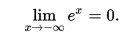The exponential function e^x is strictly increasing and has a value of 0 when in minus infinity!

We are talking about an infinite limit when the value of the limit is infinity!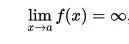The value of a can be infinity, but it doesn't have to!

The properties we talked about earlier still apply when the limit value is infinity!

In Rational functions p(x)/q(x) where p, q are polynomials we have that:

• When the degree of p is greater than the degree of q, then the limit is +- infinity.
• When the degrees of p and q are equal than the limit is equal to the division of the leading coefficients (the one in front of the largest power of x).
• When the degree of p is smaller then the degree of q then the limit equals 0.

## One-sided Limits and Continuity:

We can have x approach the limit point from the left or the right and those limits are called one-sided limits. So, when having a function f(x) and the limit when x approaches p is L we have the following two one-sided limits: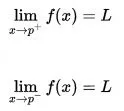This means that the limit of f when going to p from either side is p and so:

lim x->p [f(x)] = L

Sometimes, a function may don't have a limit for each side or the limit may have a different value.

When the limit values are equal for both sides then the function is continuous at that point, else we say that the function is discontinuous at that point!

Continuity:

• We say that a function is continuous at a point x0 when lim x->x0 (f(x)) = f(x0).
• When a function is continuous for all the points in a specific set then the function is continuous in that set!
• When there is at least one point for which lim x->x0 [ f(x)] != f(x0) we say that the function is discontinuous at x0 and so the point x0 is called a discontinuity point

Suppose f, g are continuous functions (or continuous at specific point) then:

• f+g, f-g, f*g are also continuous
• f/g, where g!=0 is also continuous
• |f| is also continous
• root(f) is also continuous for any root

We can also have a function be one-side continuous:

• when lim x->x0- (f(x)) = f(x0) than f is continuous from the left
• when lim x->x0+ (f(x)) = f(x0) than f is continuous from the right
• when both are true than f is (just) continuous on that point

And this is actually it and I hope you enjoyed it!

Next time we will continue with Continuity ( see what I did there? :D ) and get into some examples so that you understand it better! I just wanted to give you a basic understanding of limits today...

Bye!

H2
H3
H4
3 columns
2 columns
1 column
Join the conversation now